# Less Than Sign – Definition with Examples

## Less Than

The relationship between two quantities can be described using comparison terms. There are primarily three comparison terms: greater than (>), less than (<), and equal to (=).

Greater than (>):

When one quantity is more than the other quantity, we use “greater than”.

For example, 5 > 3.

Less than (<):

When one quantity is smaller than the other quantity, we use “less than”.

For example, 8 < 10.

Equal to (=):

When two quantities are the same, we use “equal to”.

For example, 15 = 15.

## Less than Definition

It is one of the terms used to show a relationship between two values. It states that one value is lesser than or lower than or fewer than or smaller than the other. The less than symbol is “<” and with this metric, we can compare numbers, weights, heights, and values.

Let’s look at some examples of less than.

Example 1, there are 4 marbles in Bowl A, and 7 marbles in Bowl B. On comparing the two, it is clear that Bowl A has fewer marbles than Bowl B.

Weights can be compared similarly.

Example 2, Ray buys 8 lb of potatoes and 4 lb of tomatoes and puts them in two separate bags. He hands over the lighter bag to his son. Which one does he hold himself?

Well, for that, it is important to find out which bag is lighter. There are two bags, the one with the potatoes weighs 8 lb, and the other that has tomatoes weighs 4 lb. So, the bag of tomatoes weighs less and Ray hands it to his son, while he carries the bag of potatoes.

Example 3, John has 6 bars of chocolates. Rita has 2 bars of chocolates and Suma has 3 bars of chocolates. Does John have more chocolates than Rita and Suma combined?

The total number of bars that Rita and Suma have = 2 + 3 = 5 bars

And John has 6 bars of chocolates.

Therefore, Rita and Suma together have fewer chocolates than John.

## Less Than on the Number Line

To show the lesser than sign on the number line, we use an empty circle to mark the limit value and point an arrow towards the given condition of inequality. The empty circle shows that a limit value is not the solution to the given inequality.

Let’s look at the steps to represent inequality of the form x < 3 on the number line.

Step 1: Locate “3” on the number line and mark it with the empty circle.

Step 2: The inequality x < 3 shows that the variable x is allowed to have any value smaller than 3, which means all the values on the left of this circle. Starting from the empty circle(limit value), extend an arrow to the left end signifying all the values the variable x can have.

Note: If the inequality was “less than or equal to” we would have filled the circle. A filled circle shows that the limit value is also the solution to given inequality.

## Difference between Comparison Symbols

To sum up, comparison plays a significant role in our lives. Without comparison, it is difficult to show the equality or inequality between things that we see around us.

## Solved Examples

Example 1: Select the numbers that are smaller than 20 from the given set of numbers.

0, 14, 21, 20, 18, 22, 36, 45, 12, 3, 15, 99, 27

Solution:

0, 14, 18, 12, 3, 15 – are all numbers < 20.

Example 2: In an aquarium, there are various types of fish.

Which type of fish are less than 60 in number?

Solution:

Guppies (45), zebra fish (25), and tiger barb (26) are all < 60 in number.

Example 3: Graph x < 5.

Solution:

Example 4: State whether true or false:

1.  15 < 15
2.  15 < 18
3.  15 < 14
4.  15 < 20

Solution:

1. False
2. True
3. False
4. True

## Practice Problems

1

### Choose the possible value that satisfies a number$\lt$10.

10
4
14
20
CorrectIncorrect
4$\lt$10. All other options are either greater than or equal to 10.
2

### Choose the correct graph that best describes numbers $\lt$ 4.

(a)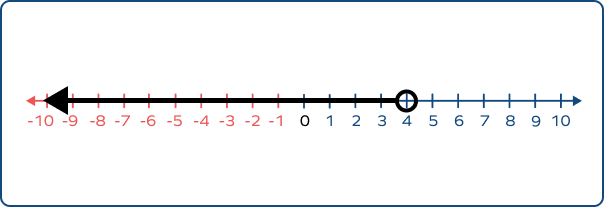(b)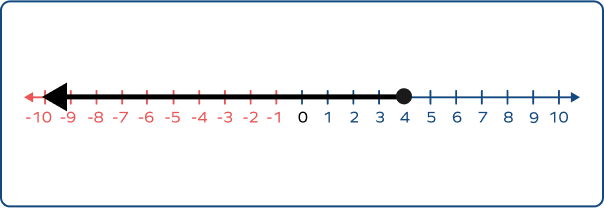(c)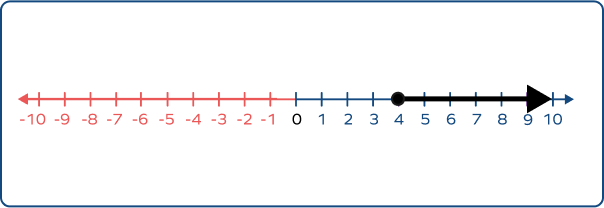(d)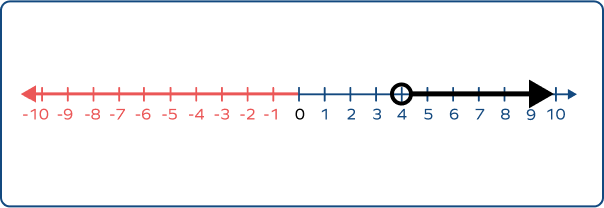CorrectIncorrect
The graph of numbers $\lt$ 4 should have an empty circle marked at 4, and an arrow starting from 4 and extending to the left of it.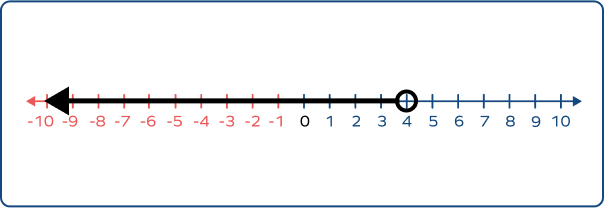3

All of them
CorrectIncorrect
42 (B) $\lt$ 44 (D) $\lt$ 46 (C) $\lt$ 50 (A). So, basket B has fewer apples.
4

### Choose the statement that explains 23 $\lt$ 50.

23 is equal to 50
23 is greater than 50
23 is less than 50
23 can be lesser than 50
CorrectIncorrect
Correct answer is: 23 is less than 50
The “$\lt$” symbol represents less than.